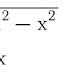Introduction:

This page is going to explain you about all the basic equations, formulas, their derivations and relations among different parameters of a simple harmonic motion. 'Simple harmonic motion' as the name also suggests, is the simplest form of an oscillatory motion.

The equations used in describing a SHM are classified in two groups viz. dynamic equations and kinematic equations. Dynamic equations are those equations which contain the information about fundamental cause (i.e. the force or torque) that actually constrained the particle to move simple harmonically. So these are basically force or torque equations. On the other hand, the measurable or observable effects produced by the dynamic factors are represented with the help of kinematic equations.

In simple terms, an equation containing 'force' or 'torque' as a variable is an equation of dynamics. And an equation which does not contain force or torque as a variable, instead includes speed, velocity, displacement, acceleration, angular acceleration etc. as their parameters, are equations of kinematics.

Dynamics of SHM

The nature of force required to set a particle in a general oscillatory motion is given by  F = -kx²ⁿ⁺¹ where n = 0, 1, 2, 3... and k is some positive constant.

The simplest possible function of force would be obtained when we put n = 0 and this will obviously be the case representing a simple harmonic motion. So a particle moving under the influence of a unidimensional force given by F = -kx will perform SHM. In case of angular SHM, the required torque is nothing but τ = -kθ.

Also since the value of x in SHM can vary from -A to +A, the magnitude of force will lie in the range of [0, kA]. In other words, the maximum possible magnitude of force in SHM is +kA and that happens when the particle is at one of the extremes (or at maximum possible distance from the mean position). The minimum possible force is zero and this occurs when the particle crosses the mean position.

Kinematics of SHM

For a particle performing simple harmonic motion its kinematic parameters of motion like displacement, velocity and acceleration are simplest periodic functions of time i.e. the sinusoidal functions of time. Therefore the time dependency of displacement, velocity and acceleration functions can be represented as follows:

x = Asin(ωt + Φ)
v = Aωcos(ωt + Φ)  and
a = -Aω²sin(ωt + Φ)

Further 'v' and 'a' as a function of 'x' can be concluded as:

Obtaining Kinematic Equations using Force Equation

In this section, we are going to show that, for a particle moving under the influence of a unidirectional force F = -kx, its kinematic parameters i.e. displacement, velocity and acceleration will hold a sinusoidal relationship with time.

Let's say a particle of mass m moves along x-axis under the influence of a unidimensional force F = - kx where x is the position coordinate of the particle. Say at the  first instant of observation i.e. at t = 0, the particle is seen at a position x = xₒ with velocity v = vₒ and at some other general instant t = t it is at x = x with velocity v = v.

Obviously ω is a positive constant and is known as angular frequency. Also from definition of acceleration we can substitute a = dv/dt = vdv/dx.
Hence, whenever a particle is acted upon by a restoring force of nature F = - kx, its displacement, velocity and acceleration vary sinusoidally with time.

Obtaining Force Equation using Kinematic Equations

Let's now do the reverse of the previous case. We'll start from x = Asin(ωt + Φ) and end up with the equation F = -kx.

x = Asin(ωt + Φ)           ...1
⇒ v = Aωcos(ωt + Φ)       ... 2
⇒ a = -Aω²sin(ωt + Φ)     ... 3
Equation number 3 can be rewritten as
a = -ω²Asin(ωt + Φ)
⇒ a = - ω²x                       ... 4
[using eq. 1]
⇒ F/m = - ω²x
⇒ F = -(mω²) x
Here (mω²) = +ve constant = k (say)
∴    F = -kx

Dynamics and Kinematics of SHM: Basic Equations & DerivationsReviewed by Raushan Raj on July 29, 2019 Rating: 5